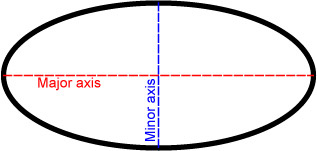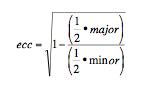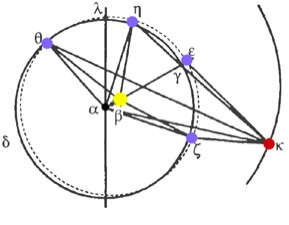Introduction:

This lesson is designed for middle school students with no previous knowledge of ellipses. This lesson easily follows the "Brahe, Kepler, and Galileo" lesson as it elaborates on the properties of ellipses. Students are expected to do some investigations and discoveries as a part of this lesson, so the time required may vary depending on your students.

Any

Learning Objectives:

• Define ellipse and label the parts of an ellipse
• Describe the properties of ellipses
• Explain Kepler's Laws of Planetary Orbit
• Understand how the eccentricity of Earth affects the planet

Guiding Question:

Why was the Scientific Revolution important and how did it contribute to progress?

Materials:

Images, cardboard, thumbtacks/push pins, pencils, string tied in loops.

Books:

• The Story of Science Newton at the Center by Joy Hakim. Published by Smithsonian Books, 2005. (pp. 129-131)
Websites:

• Kepler's Discovery is a fantastic online resource that breaks down clearly and succinctly the process of Kepler's discovery that planetary orbits are elliptical. The site contains some excellent animations and brief histories of astronomers.

Procedure:

[Note: This lesson in its entirety can be found as an attached pdf and doc file.]

Lesson Summary:

• Introduce the definition of an ellipse
• Draw ellipses
• Discover fundamental properties of ellipses
• Develop an understanding of eccentricity
• Review the impact of ellipses on planetary orbits as stated in Kepler's Laws of Planetary Motion
• Introduce properties of Earth's eccentricity

Lesson: ellipses

[Start this lesson with a cone made of Styrofoam, jell-o, model magic, or anything else that’s solid, but malleable.]

What is an Ellipse?
An ellipse is not a circle, and it’s not an egg. It’s kind of like an oval, but not quite. Strictly speaking, it’s an elongated oval.

If you imagine (or have) a cone and you cut a slice of the cone that’s parallel to the base, what shape do you get? [Do this so the students can see that you would get a circle] But, what if you make a slice that’s not parallel to the base? [Also make this cut] A non-parallel slice of cone produces an ellipse. But, you don’t need a cone to make an ellipse, they’re actually fairly simple to draw.

[Each student needs a piece of cardboard, some sheets of white paper that fit on top of the cardboard, two tacks, a string tied into a loop, and a pencil]

Procedure:

1. Lay one piece of paper on top of the cardboard
2. Stick the two pushpins in the cardboard a few inches from each other
3. Loop the string around both tacks and your pencil, so that if you were to pull the string tight it would make a triangle whose points are the two tacks and the pencil
4. Place the pencil on the cardboard, so that the string is taught and trace the pencil along the inside of the loop of string until your pencil is back where you began
5. You should now have an ellipse on the paper

Move the two tacks farther or closer apart and draw another ellipse.

How do the two ellipses compare? [Have students share their observations with one another.]

If you put the tacks on top of each other (or simply use one tack), what shape results? [Students should draw a circle]

All ellipses have two fixed points inside, those are the tacks, they’re called foci.

Investigating Properties of Ellipses
Now, mark any spot on the perimeter. Take a ruler, and measure the distance from that point to each of the foci. Add those distances up.

Now choose a different point on the perimeter and do it again.

Is the total the same? [Students should notice that the sum is the same each time for everyone in the class] It will always be the same no matter which point of the perimeter you choose.

In the case of the Earth’s elliptical orbit of the Sun, the Sun isn’t at the center; it’s at a focus. It was Kepler who found that this is true for every planet!

Does the diameter of a circle ever change? [No] How do you measure the diameter? [Measure a line through the center of the circle] How would we measure the diameter of an ellipse? [Students may have a variety of answers for this]

An ellipse’s diameter varies in length. The longest diameter is called the major axis; the shortest is the minor axis.(Author: Meredith Beaton License: Public Domain)

The flatter the ellipse, the greater the difference between the major axis and the minor axis. So, if an ellipse has a very small difference between the major and minor axis, what shape is it likely to resemble? [A circle]

There’s a special name for that difference between the major and minor axis. Mathematicians call it the eccentricity. Essentially, the eccentricity of an ellipse tells you just how flat the ellipse is.

Mathematicians have a special way of calculating the eccentricity because they like it to be a positive number between zero and one. So, the official way of calculating the difference is:The two foci are always on the major axis of an ellipse. Draw the major and minor axes on two of your ellipses, and try to be as precise as possible.

Now, measure the distance from each foci to the center of the ellipse—the center is where the major and minor axes intersect. What did you find? [Students should find that the distance from each focus to the center is the same. If students are finding their numbers to a be a bit off, this is a great time to have a quick impromptu discussion about human error.]

In fact, for all ellipses, each focus will be the same distance from the center of the ellipse!

Thanks to Kepler
Although Kepler lived a somewhat tragic life, his contributions were some of the most important evidence that the Copernican Model was correct. Kepler’s three laws accurately described the way planets moved, and we still rely on them today to predict planetary motion. Kepler found:

1. Each planet travels around the Sun in a path that is an ellipse. The Sun is located at one of the foci, so as the planet travels in its obit its distance from the Sun changes.
2. The speed of a planet’s orbit depends on its distance from the Sun—the close a planet is to the Sun, the faster it travels, and the farther a planet it from the Sun, the slower it travels.
3. The farther a planet is from the Sun, the longer its orbit of the Sun will take, and the longer its year will last.

Earth’s Eccentricity
Earth’s eccentricity is about 0.017, meaning that at its farthest point from the Sun it’s about 3 million miles farther from the Sun than it is at its closest point to the Sun. That might sound like a lot, but in fact it’s quite small. If we look at a picture of Earth’s obit, you see that it actually looks very circular. In fact, you need very precise measuring tools to identify that Earth’s orbit is an ellipse.(Kepler's original illustration of Earth's predicted orbit--solid circle--versus his observed orbit of Earth--dotted circle. From New Astronomy by Johannes Kepler. License: Public Domain)
What’s especially interesting is that the Earth’s orbit actually changes eccentricity. Every 100,000 years it cycles from nearly circular to more elliptical and back. During years when the Earth is more elliptical, and therefore more eccentric, the Earth is farther from the Sun and closer to the Sun than during times of nearly circular orbit. This means that the Earth experiences greater variation in the amount of solar energy that reaches us, and this variation has a direct effect on our climate!

Assessment:

Students are asked to answer a series of short answer questions. The assessment can be found as a separate wiki page here, where there is also a pdf and doc version available for download.

Attached Files:

Non-profit Tax ID # 203478467